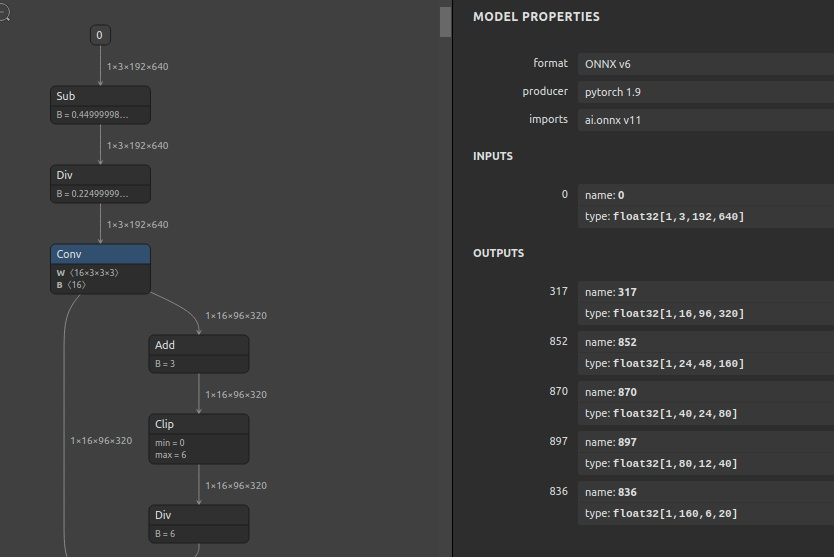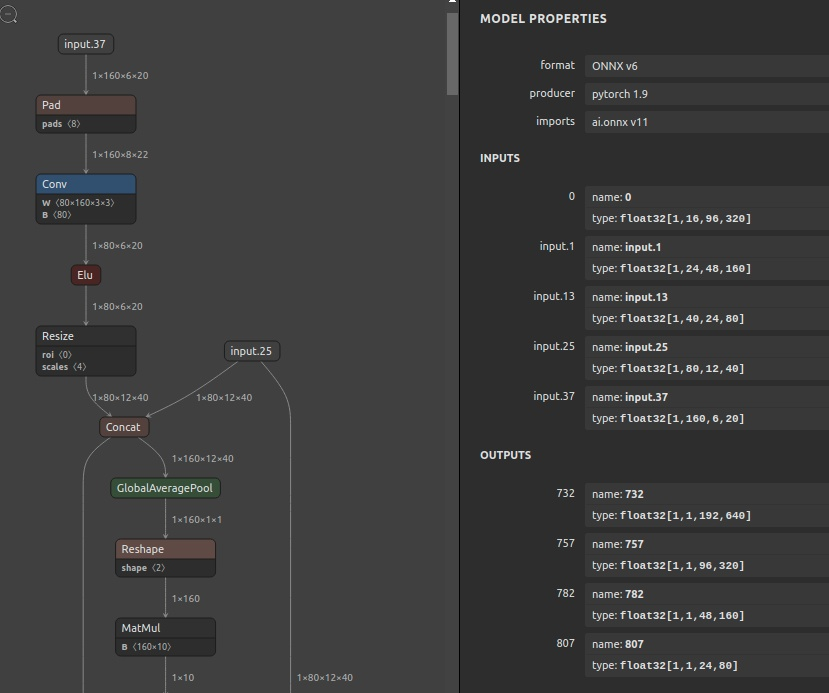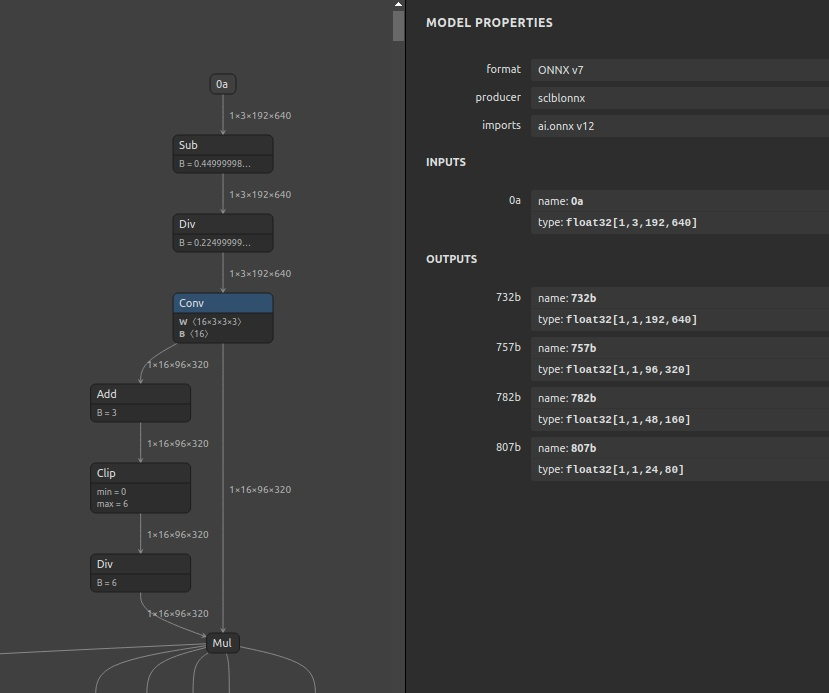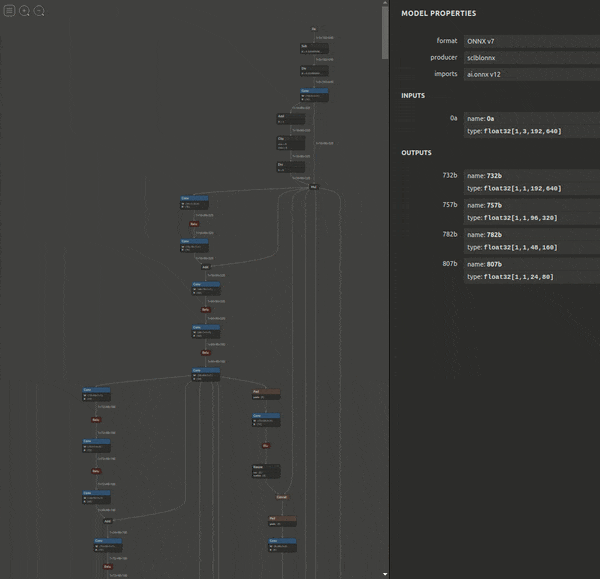🤪

# Steps to merge two ONNX files into one

2021/09/27に公開約4,100字

# 1. Introduction

This section describes a simple procedure for combining two ONNX into one ONNX. Because it's a pain to modify the original PyTorch program when exporting ONNX from PyTorch. In order to combine two ONNX with this simple procedure, the prerequisites are

1. The number of outputs of the first ONNX must match the number of inputs of the second ONNX.
2. The shape of the output of the first ONNX must match the shape of the input of the second ONNX.
• `ONNX.1: lite_hr_depth_k_t_encoder_192x640.onnx`

• `INPUT: ['0']`
• `OUTPUT: ['317', '852', '870', '897', '836']`• `ONNX.2: lite_hr_depth_k_t_depth_192x640.onnx`

• `INPUT: ['0', 'input.1', 'input.13', 'input.25', 'input.37']`
• `OUTPUT: ['732', '757', '782', '807']`• `Result ONNX (ONNX.1 + ONNX.2): lite_hr_depth_k_t_encoder_depth_192x640.onnx`

• `INPUT: ['0a']`
• `OUTPUT: ['732b', '757b', '782b', '807b']`# 2. Environment

1. Python3.x
2. ONNX 1.8.x
3. onnx-graphsurgeon
4. sclblonnx

# 3. Procedure

## 3-1. Install

``````\$ python3 -m pip install onnx_graphsurgeon \
--index-url https://pypi.ngc.nvidia.com
\$ pip install sclblonnx
``````

## 3-2. Merge

1. Create `merge_onnx.py`.
• The operation name of the first ONNX must not overlap with the operation name of the second ONNX at all, so we rename them in the program to never overlap.
• Force the `a` prefix to the end of the first ONNX operation name.
• Force the `b` prefix to the end of the second ONNX operation.
• Modify the name of the operation with `onnx_graphsurgeon`.
• Combine two ONNX with `sclblonnx`
``````import onnx
import onnx_graphsurgeon as gs
import sclblonnx as so

H=192
W=640
MODEL1=f'lite_hr_depth_k_t_encoder_{H}x{W}.onnx'
MODEL2=f'lite_hr_depth_k_t_depth_{H}x{W}.onnx'
MODEL3=f'lite_hr_depth_k_t_encoder_depth_{H}x{W}.onnx'

for n in graph1.nodes:
for cn in n.inputs:
if cn.name[-1:] != 'a':
cn.name = f'{cn.name}a'
else:
pass
for cn in n.outputs:
if cn.name[-1:] != 'a':
cn.name = f'{cn.name}a'
else:
pass
graph1_outputs = [o.name for o in graph1.outputs]
print(f'graph1 outputs: {graph1_outputs}')
onnx.save(gs.export_onnx(graph1), "graph1.onnx")

graph2_inputs = []
for n in graph2.nodes:
for cn in n.inputs:
if cn.name[-1:] != 'b':
cn.name = f'{cn.name}b'
else:
pass
for cn in n.outputs:
if cn.name[-1:] != 'b':
cn.name = f'{cn.name}b'
else:
pass
graph2_inputs = [i.name for i in graph2.inputs]
print(f'graph2 inputs: {graph2_inputs}')
onnx.save(gs.export_onnx(graph2), "graph2.onnx")

"""
graph1 outputs: [
'317a',
'852a',
'870a',
'897a',
'836a'
]
graph2 inputs: [
'0b',
'input.1b',
'input.13b',
'input.25b',
'input.37b'
]
"""
sg1 = so.graph_from_file('graph1.onnx')
sg2 = so.graph_from_file('graph2.onnx')
sg3 = so.merge(
sg1,
sg2,
outputs=graph1_outputs,
inputs=graph2_inputs
)

so.graph_to_file(sg3, MODEL3)
``````
1. Run `merge_onnx.py`.
``````\$ python3 merge_onnx.py

graph1 outputs: ['317a', '852a', '870a', '897a', '836a']
graph2 inputs: ['0b', 'input.1b', 'input.13b', 'input.25b', 'input.37b']
Constructing the io_match list from your input and output.
``````# 4. Appendix

https://github.com/NVIDIA/TensorRT/tree/master/tools/onnx-graphsurgeon
https://github.com/scailable/sclblonnx
https://github.com/shawLyu/HR-Depth
https://github.com/PINTO0309/PINTO_model_zoo/tree/main/158_HR-Depth

### Discussionログインするとコメントできます